• python画散点图问题描述实例数据准备绘制散点图结果展示参考 问题描述 总结一个普遍的画图格式太难了，我就举一个比较复杂的例子来说明，比如我有一个嵌入向量矩阵(N*128)，想将其可视化到二维平面，所以就用t-sne将...
python画散点图问题描述实例数据准备绘制散点图结果展示参考
问题描述
总结一个普遍的画图格式太难了，我就举一个比较复杂的例子来说明，比如我有一个嵌入向量矩阵(N*128)，想将其可视化到二维平面，所以就用t-sne将其降维并画出散点图。该嵌入向量表示的点有两类，并且希望不同类别的点在散点图上具有不同的大小、形状、颜色和透明度。
实例
数据准备

有一个（N**2）的数组（ndarray/list），和一个N*1的list来说明每个点的类型

import numpy as np
from sklearn.decomposition import PCA
from sklearn.manifold import TSNE
import matplotlib.pyplot as plt
import pandas as pd
'''
把高维数据通过t-sne降维成二维的数据
'''
addr = r"addr"
embedding_addr = addr + r"\embedding.txt"
label_addr = addr + r"\label.txt"
embedding = np.loadtxt(embedding_addr) #N*128
label = np.loadtxt(label_addr) #N
newX = TSNE(n_components=2,random_state=33).fit_transform(embedding)#(N*2)



将其根据类型拆分成两个数据集data1,data2

type1_x = []
type1_y = []
type2_x = []
type2_y = []
for i in range(len(label)-8700):#为了图清晰一些，故意删去了一些点
#if i not in test_dataset_index:
if label[i]==0:
type1_x.append(newX[i])
type1_y.append(newX[i])
if label[i]==1:
type2_x.append(newX[i])
type2_y.append(newX[i])
#转化为ndarray类型
type1_x = np.array(type1_x)
type1_y = np.array(type1_y)
type2_x = np.array(type2_x)
type2_y = np.array(type2_y)
#data1,data2 分别是 N1*2，N2*2
data1 = np.column_stack((type1_x,type1_y)) #把x坐标和y坐标拼接起来
data2 = np.column_stack((type2_x,type2_y)) #把x坐标和y坐标拼接起来


设置两种数据集描述的点在散点图中的特点，比如颜色、大小以及形状

type1_color = 'green'
type2_color = 'red'
type1_size = 20
type2_size = 40
type1_marker = 's'
type2_marker = 'p'
type1_transparent = 0.3
type2_transparent = 1


其中color的可选项有（简写和全拼都可）
‘b‘= blue(蓝色)
‘g’=green(绿色)
‘r’=red(红色)
‘c’=cyan(青色)
‘m’=magenta(品红)
‘y’=yellow(黄色)
‘k’=black(黑色)
‘w’=white(白色)
大小size的默认值是20，可调
其中形状的可选项有透明度是从0~1

DataFrame这两个数据集

df1 = pd.DataFrame(data1, columns=['x','y'])
df2 = pd.DataFrame(data2, columns=['x','y'])

绘制散点图
'''
每一类数据依次在图上画出来
kind = scatter 意为散点图
x，y是指横纵坐标
c = color
s = size
label 图注
'''
ax = df1.plot(kind='scatter', x='x', y='y', c=type1_color,s=type1_size, marker=type1_marker,alpha=type1_transparent,label="type1")
df2.plot(kind='scatter', x='x', y='y', c=type2_color,s=type2_size, marker=type2_marker,alpha=type2_transparent,ax=ax,label="type2")#两个类别依次画在图上

plt.show()

结果展示参考

https://blog.csdn.net/qq_40949544/article/details/88222538
https://www.gairuo.com/p/pandas-plot-scatter
https://www.cnblogs.com/shanger/p/12925703.html
https://blog.csdn.net/h_hxx/article/details/90635650


展开全文可视化 pandas
• Python 画散点图 # 2020.01.06 By yangbocsu 参考：https://blog.csdn.net/w576233728/article/details/86538060 import numpy as np import matplotlib.pyplot as plt #matplotlib画图中中文显示会有问题，需要...
Python 画散点图

版本一：

# 2020.01.06  By yangbocsu  参考：https://blog.csdn.net/w576233728/article/details/86538060
import numpy as np
import matplotlib.pyplot as plt

#matplotlib画图中中文显示会有问题，需要这两行设置默认字体
plt.rcParams['font.sans-serif']=['SimHei']
plt.rcParams['axes.unicode_minus'] = False

#画的坐标轴并设置轴标签x，y
plt.xlabel('X')
plt.ylabel('Y')
plt.xlim(xmax=12,xmin=0)
plt.ylim(ymax=12,ymin=0)

#散点 数据
x1=np.arange(1,10,1)
y1=np.array([1,3,3,3,5,6,7,8,10])

area = np.pi * 2**2  # 点面积

plt.scatter(x1, y1, s=area, alpha=0.4,color="red", label='类别A')

plt.legend() #label='类别A'
plt.savefig(r'C:\Users\97942\Desktop\hw1_data\12345svm.png', dpi=600)
plt.show()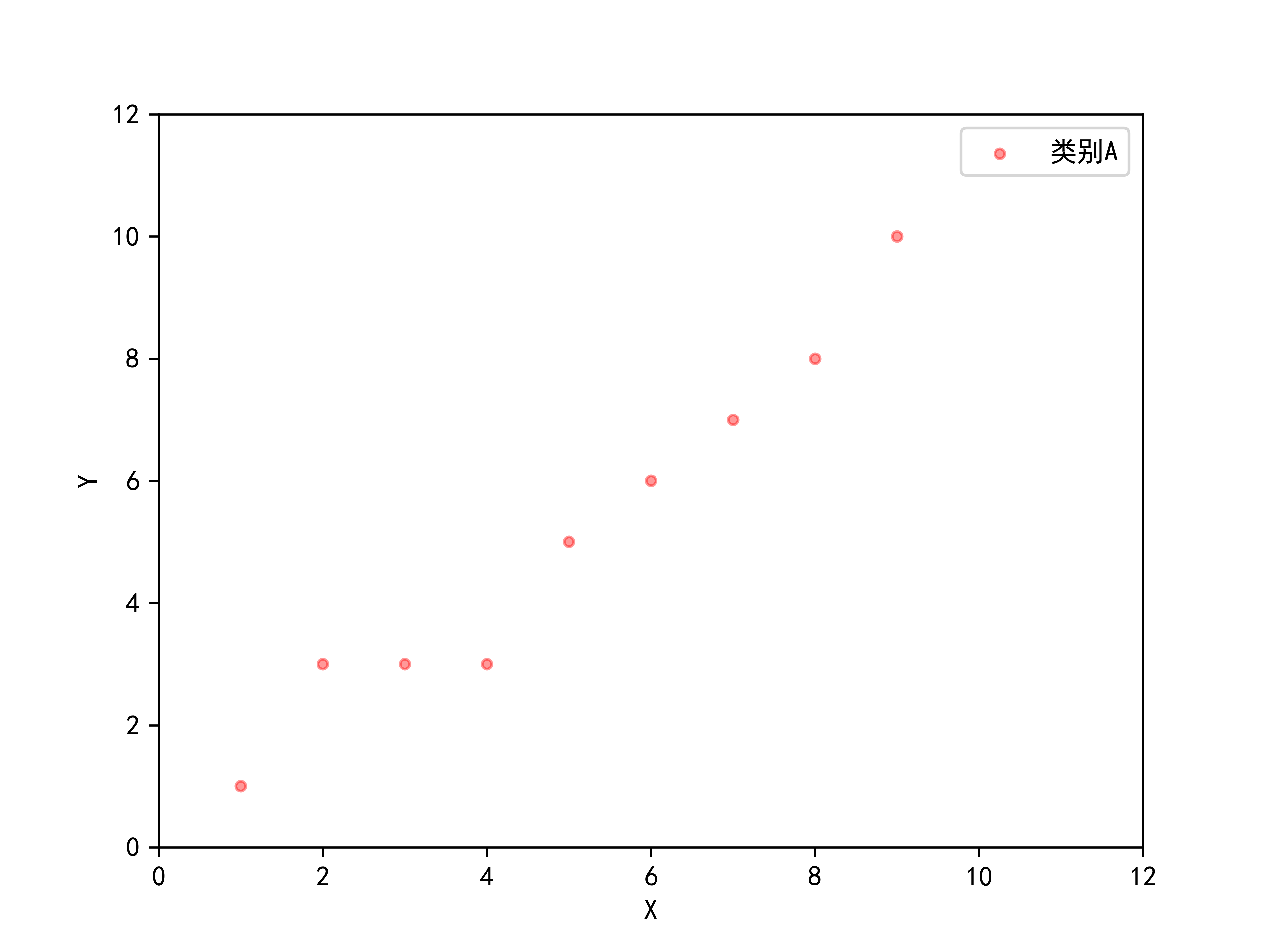版本二：

# 2020.01.06  By yangbocsu
import numpy as np
import matplotlib.pyplot as plt

#matplotlib画图中中文显示会有问题，需要这两行设置默认字体
plt.rcParams['font.sans-serif']=['SimHei']
plt.rcParams['axes.unicode_minus'] = False

#画的坐标轴并设置轴标签x，y
plt.xlabel('X')
plt.ylabel('Y')
plt.xlim(xmax=12,xmin=0)
plt.ylim(ymax=12,ymin=0)

#散点图数据
x1=np.arange(1,10,1)
y1=np.array([1,3,3,3,5,6,7,8,10])

plt.scatter(x1, y1, marker = 'o', alpha=0.4,color="red", label='类别A')

plt.legend() #label='类别A' 图中显示
plt.title('用pyhon画散点图')#图的标题

plt.savefig(r'C:\Users\97942\Desktop\hw1_data\12345svm.png', dpi=600)
plt.show()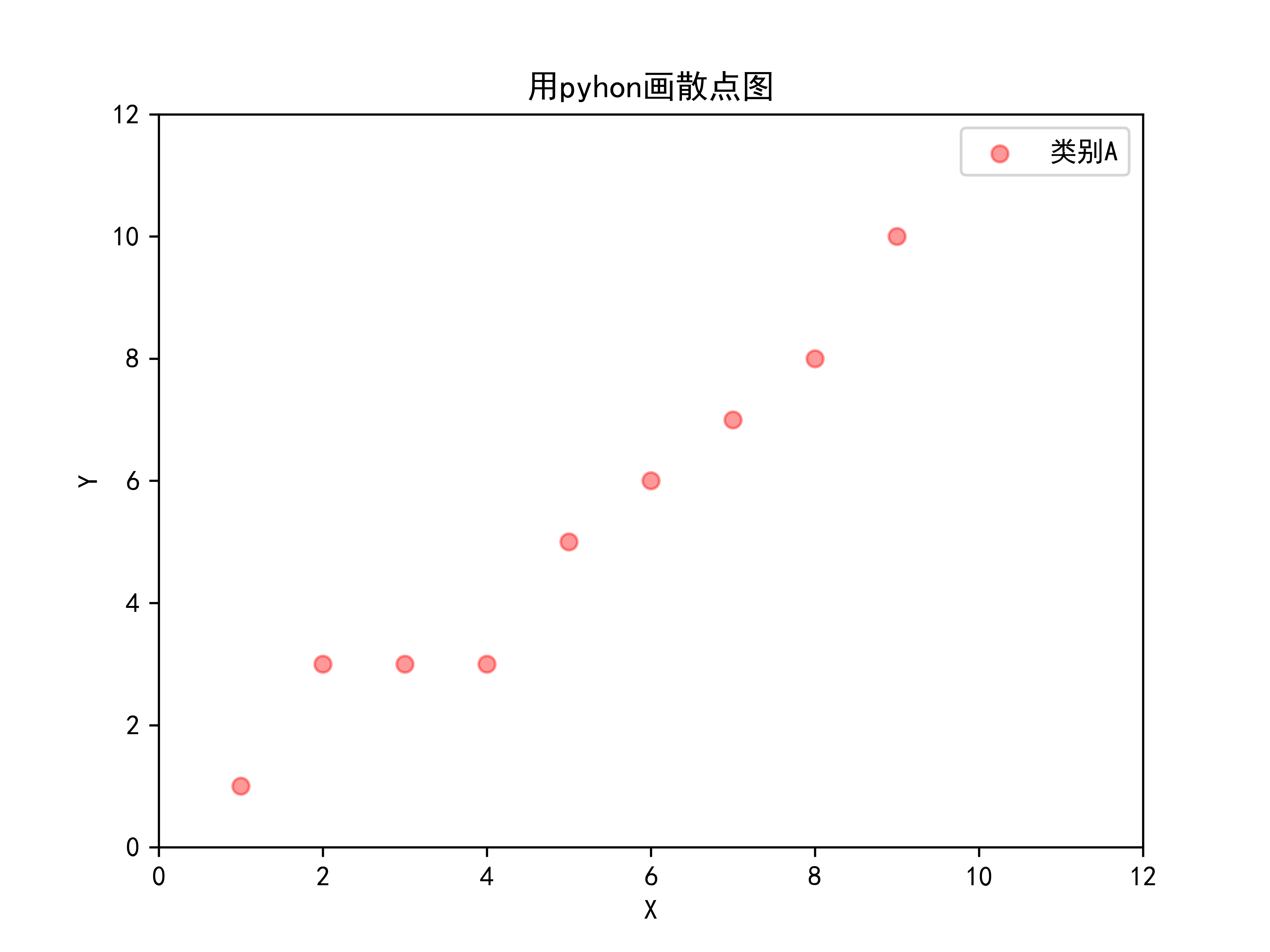展开全文• matplotlib模块的pyplot有画散点图的函数，但是该函数要求x轴是数字类型。 pandas的plot函数里，散点图类型scatter也要求数字型的，用时间类型的会报错。 在搜索阅读了几十篇网文后，摸索出画散点图的简单办法。 ...广告关闭腾讯云11.11云上盛惠 ，精选热门产品助力上云，云服务器首年88元起，买的越多返的越多，最高返5000元！用散点图可以直观的查看数据的分布情况。 matplotlib模块的pyplot有画散点图的函数，但是该函数要求x轴是数字类型。 pandas的plot函数里，散点图类型scatter也要求数字型的，用时间类型的会报错。 在搜索阅读了几十篇网文后，摸索出画散点图的简单办法。 可以使用pyplot的plot_date()画散点图。 下面是完整的python...我现在有一个nx3矩阵数组。 我想把这三列画成三轴，我怎么能这样做呢？ 我在谷歌上搜索过，人们建议用matlab但是我真的很难理解它。 我还需要一个散点图。 有人能教我吗？...关联图是查找两个事物之间关系的图像，他能为我们展示出一个事物随着另一个事物的变化如何变化。 典型的关联图有：折线图、散点图、相关矩阵等我们什么时候会需要关联图？ 1、数据报告 & 学术研究展示趋势：比如产品销量随着时间如何变化，智力水平随着教育程度如何变化等展现状态：不同年龄的客户的成交率...下面是一个制作散点图动画的例子：import plotly.express as pxdf = px.data.gapminder()fig =px.scatter( df, x=gdppercap, y=lifeexp, animation_frame=...而漂漂亮亮的可视化是完成这一任务的绝佳工具。 本文将介绍 5 种非传统的可视化技术，可让你的数据故事更漂亮和更有效。 这里将使用 python 的 plotly ...下面是一个制作散点图动画的例子：import plotly.express as pxdf = px.data.gapminder()fig =px.scatter( df, x=gdppercap, y=lifeexp, animation_frame=...而漂漂亮亮的可视化是完成这一任务的绝佳工具。 本文将介绍 5 种非传统的可视化技术，可让你的数据故事更漂亮和更有效。 这里将使用 python 的 plotly ...来自维基百科不过，我们仍然可以绘制出多维空间，今天就来用 python 的 plotly 库绘制下三维到六维的图，看看长什么样。 数据我们使用一份来自 uci 的真实汽车数据集，该数据集包括 205 个样本和 26 个特征，从中选择 6 个特征来绘制图形：? 基础工作安装好 plotly 包：pip install plotly加载数据集（文末会提供）...今日锦囊常用的统计图在python里怎么画? 这里的话我们介绍几种很简单但也很实用的统计图绘制方法，分别有条形图、饼图、箱体图、直方图以及散点图，关于这几种图形的含义这边就不多做解释了。 今天用到两个数据集，数据集大家可以在公众号回复特征工程来获取，分别是salary_ranges_by_job_classification和globalland...下面，我们使用python画一些plolty基本图的demo：（大家如果有需要用plotly的建议收藏哦！ 散点图散点图核心的价值在于发现变量之间的关系，千万不要简单地将这个关系理解为线性回归关系。 变量间的关系有很多，如线性关系、指数关系、对数关系等等，当然，没有关系也是一种重要的关系。 散点图更偏向于研究型图表...选自towardsdatascience作者：william koehrsen机器之心编译参与：nurhachu null、路 本文介绍了如何在 python中利用散点图矩阵（pairs plots）进行数据可视化。 如何快速构建强大的探索性数据分析可视化当你得到一个很不错的干净数据集时，下一步就是探索性数据分析（exploratory data analysis，eda）。 eda 可以...与线型图类似的是，散点图也是一个个点集构成的。 但不同之处在于，散点图的各点之间不会按照前后关系以线条连接起来。 用plt.plot画散点图? 奇怪，代码和前面的例子差不多，为什么这里显示的却是散点图而不是sin曲线呢？ 原因有二：一是点集比较少，稀疏，才30个； 二是没有指定线型。 用plt.scatter画散点图scatter...使用matplotlib画散点图步骤，第一种使用scatter(x,y),系统自动建立坐标系，第二种使用plot(x,y)系统也是自动建立坐标系，plot函数默认画连线图。 比较，scatter比plot适合画散点图。 7、 pandas是一个为解决python数据分析而用的包，可以快速构建数据结构。 8、scikit-learn简称sklearn,在导入数据包时只能使用...下面是一个制作散点图动画的例子：import plotly.express as pxdf = px.data.gapminder()fig =px.scatter( df, x=gdppercap, y=lifeexp, animation_frame=...而漂漂亮亮的可视化是完成这一任务的绝佳工具。 本文将介绍 5 种非传统的可视化技术，可让你的数据故事更漂亮和更有效。 这里将使用 python 的 plotly ...画散点图：# -*- coding:utf-8 -*-import pandas as pdimport numpy as npfrom sklearn.decompositionimport pcafrom sklearn.linear_model import logist...plt.grid(true)##画出虚线方格 plt.show()? 我们可以绘制在三维空间的正态分布图代码如下#! usrbinpython# -*- coding:utf-8 -*-import numpy as npfrom ...案件回顾饭团销售额下滑现有冰激凌店一年的历史销售数据数据包括单日的销售量、气温、周几（问题：如何用这些数据预测冰激凌的销量？ 模拟实验与分析将数据存储为csv格式，导入python。 并画出散点图，观察气温和销售量的关系。 import pandas as pdicecream = pd.read_csv(icecream.csv)importmatplotlib.pyplot as ...一般分三步：（1）画散点图，找模型； （2）进行回归模型的参数估计； （3）检验前面分析得到的经验模型是否合适。 画散点图创建一个datatemp的文件夹...上一篇文章讲了最小二乘算法的原理。 这篇文章通过一个简单的例子来看如何通过python实现最小乘法的线性回归模型的参数估计。 王松桂老师《线性统计模型—...2.pngdef print_scatter_draw(): 画散点图 return:x=np.random.rand(1000) y=np.random.rand(len(x)) #绘图plt.scatter(x,y,color=b,label=scatter draw, ...5.pngcopy的文章 数据挖掘之matplotlib入门发现python的代码粘贴过去，因为缩进，需要重新修改，有点麻烦 百度云 源码分享，下载下来，体验运行吧。? 图像...datinglabels = file2matrix(datingtestset2.txt) import matplotlibimportmatplotlib.pyplot as pltfig = plt.figure()ax =fig.add_subplot(111)ax.scatter(datingdatamat, datingdatamat, 15.0*array(datinglabels),15.0*array(datinglabels)) #scatter函数是用来画散点图的plt.show() 结果显示 1-4. 准备数据： ...datinglabels = file2matrix(datingtestset2.txt) 1 import matplotlib2 importmatplotlib.pyplot as plt3 fig = plt.figure()4 ax = fig.add_subplot(111)5ax.scatter(datingdatamat, datingdatamat, 15.0*array(datinglabels),15.0*array(datinglabels)) #scatter函数是用来画散点图的6 plt.show()结果显示?...流程图如下：扩容python 会根据散列表的拥挤程度扩容。 “扩容”指的是:创造更大的数组，将原有内容拷贝到新数组中。 接近 23 时，数组就会扩容。 根据键查找“键值对”的底层过程我们明白了，一个键值对是如何存储到数组中的，根据键对象取到值对象，理解起来就简单了。 a.get(name)gaoqi当我们调用 a.get(“name”)...画散点图如下：# 散点图from pylab import *n = 1024x = np.random.normal(0,1,n)y =np.random.normal(0,1,n)scatter(x,y)show()显示： ? 画条形图如下：#条形图from pylab import *n = 12x = np.arange(n)y1 = (1-xfloat(n)) *np.random.uniform(0.5,1.0,n)y2 = (1-xfloat(n)) *np.random.uniform(0.5,1.0,n)bar(x, ...
展开全文• ## python画散点图

千次阅读 2019-04-16 18:09:41
大家平时为了直观地显示数据的分布情况，在画散点图的时候，简单地把数据点用圆点标出来，像这样： 然而今天我想给大家展示的散点图，或许没有那么直观地反映数据的分布情况，不够实用，但是真的很美很艺术，每...
大家平时为了直观地显示数据的分布情况，在画散点图的时候，简单地把数据点用圆点标出来，像这样：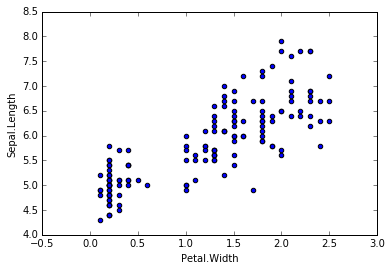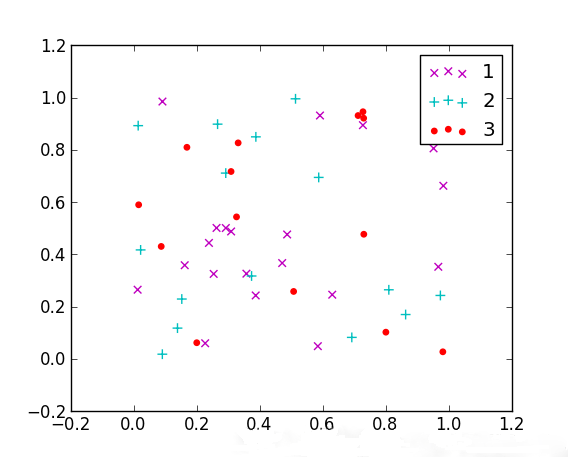然而今天我想给大家展示的散点图，或许没有那么直观地反映数据的分布情况，不够实用，但是真的很美很艺术，每个的点的颜色和大小都不一样，形状也是多变的。比如五角星的散点图：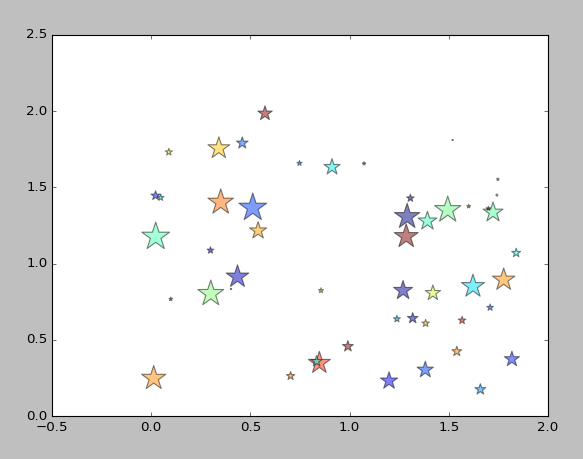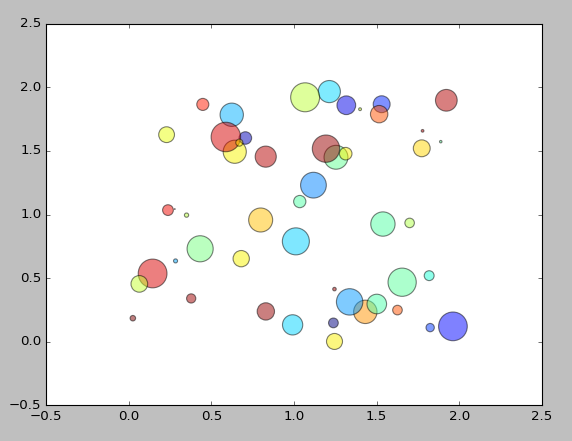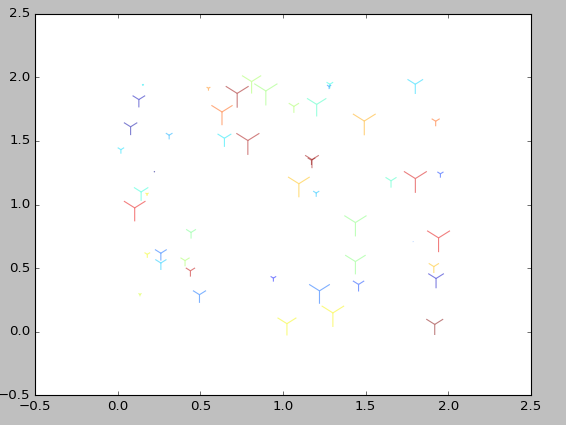等等还有很多，那么这些漂亮的形状是怎么画出来的呢？其实代码很简单：

from numpy import *;

N = 50 # 点的个数
x = np.random.rand(N) * 2 # 随机产生50个0~2之间的x坐标
y = np.random.rand(N) * 2 # 随机产生50个0~2之间的y坐标
colors = np.random.rand(N) # 随机产生50个0~1之间的颜色值
area = np.pi * (15 * np.random.rand(N))**2  # 点的半径范围:0~15
# 画散点图
plt.scatter(x, y, s=area, c=colors, alpha=0.5, marker=(9, 3, 30))
plt.show()


这里用到一个matplotlib.pyplot子库中画散点图的函数
matplotlib.pyplot.scatter(x, y, s=20, c=None, marker='o',
cmap=None, norm=None, vmin=None, vmax=None, alpha=None,
linewidths=None, verts=None, edgecolors=None, hold=None,
data=None, **kwargs）
这个函数接收的参数很多，有默认值的平时也不需要我们指定，是可选的，这次我们用到的除了基本的x ，y参数，还有c，s，alpha和marker，c就是为点指定的颜色数组，s是点的面积大小，alpha是点的颜色的透明度，marker是指定点标记的形状。在例子里指定透明度为0.5，c和s是随机生成的，我们要改变的是marker的值，marker有很多值可供选择，下表展示了在例子代码的基础上，改变marker的值后的效果：

marker
result
”.”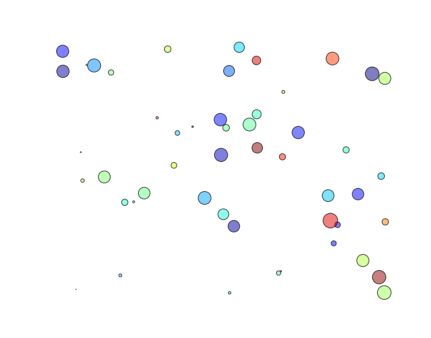”,”“o”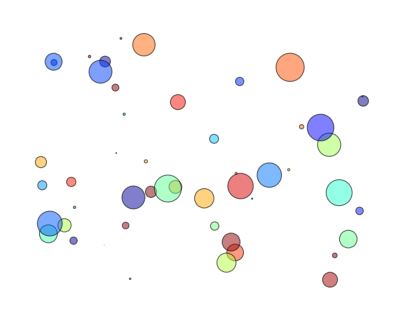“v”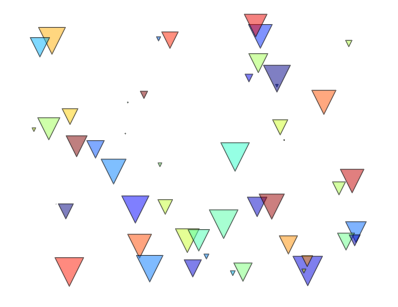“^”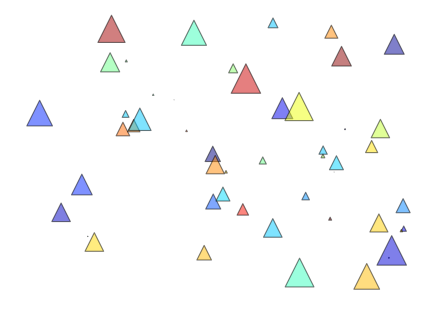“<”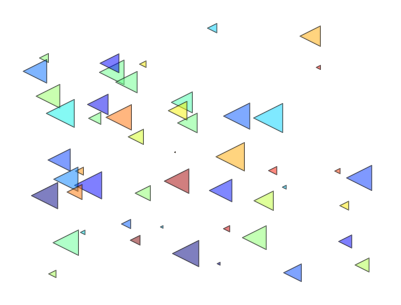“>”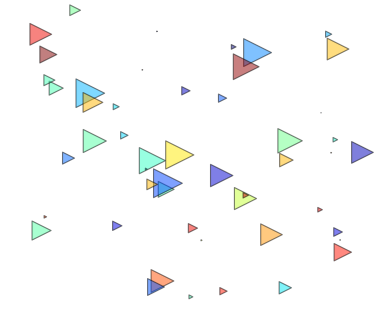“1”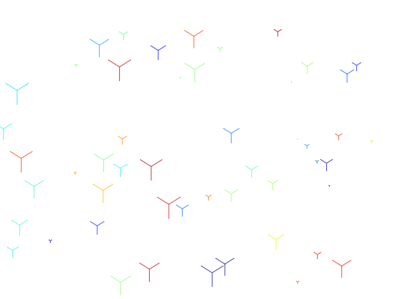“2”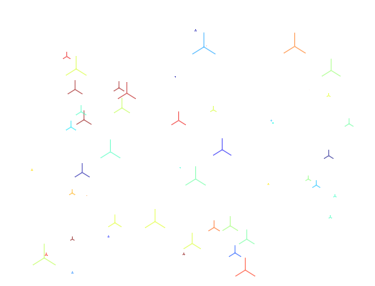“3”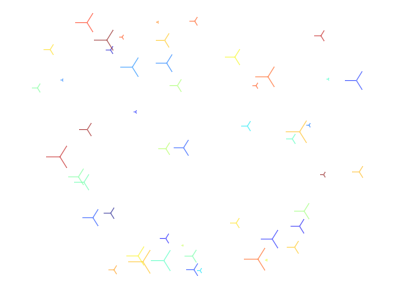“4”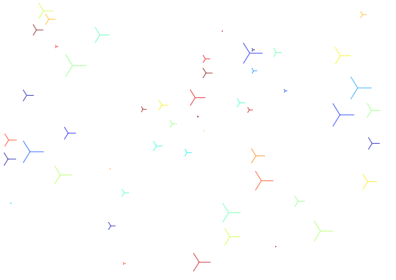“8”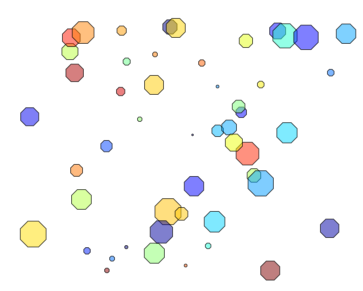“s”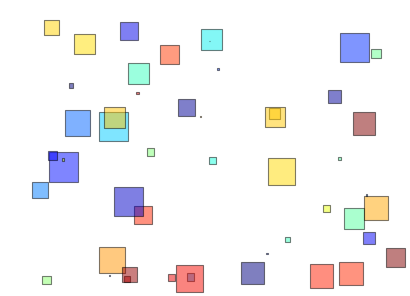“p”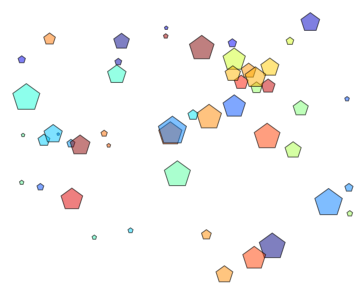“*”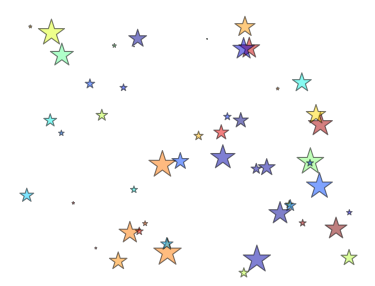“h”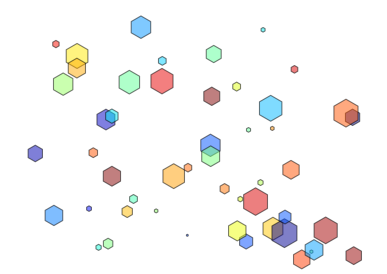“H”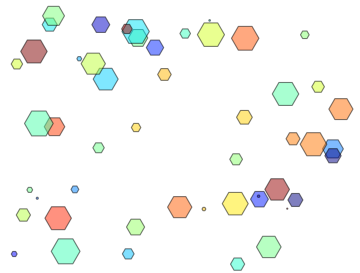“+”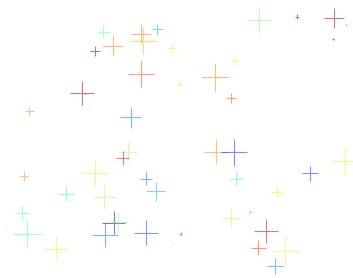“x”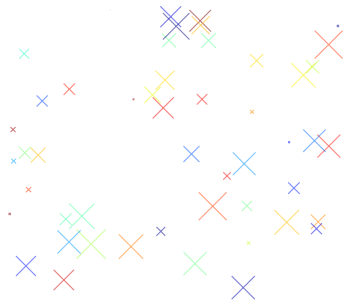“D”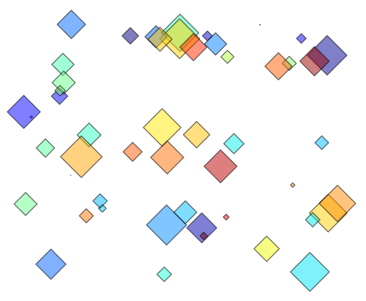“d”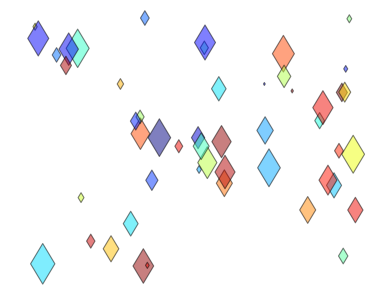“_”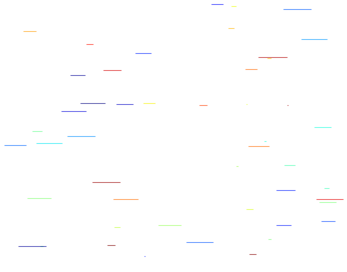“$…$”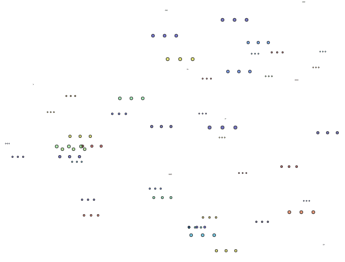(numsides, style, angle)
eg:(9,0, 30)注：numsides是边的个数，
angle是旋转角度，
style只有0,1,2,3四个值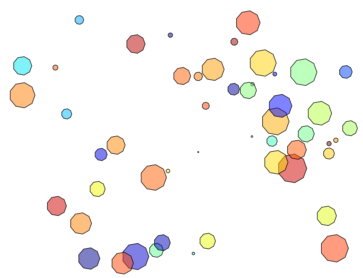(numsides, style, angle)
eg:(9,1, 30)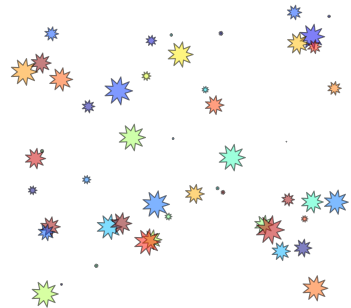(numsides, style, angle)
eg:(9,2, 30)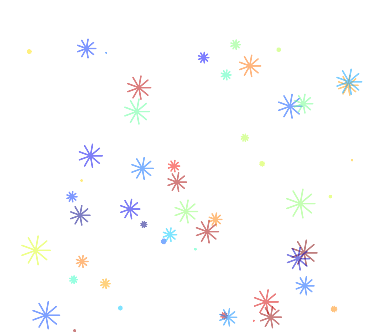(numsides, style, angle)
eg:(9,3, 30)注：此时numsides和angle的值自动被忽略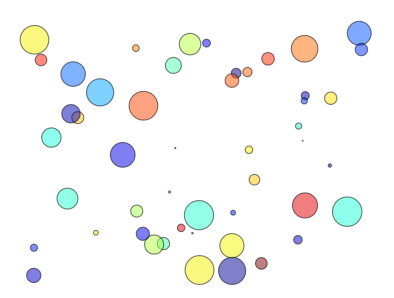展开全文• ## Python画散点图

千次阅读 2020-07-19 18:12:42
import matplotlib.pyplot as plt import numpy as np import pandas as pd my_dpi=96 plt.figure(figsize=(480/my_dpi, 480/my_dpi), dpi=my_dpi) df=pd.DataFrame({'x': range(1,101), 'y': np.random.randn(100...
• matplotlib模块的pyplot有画散点图的函数，但是该函数要求x轴是数字类型。pandas的plot函数里，散点图类型"scatter"也要求数字型的，用时间类型的会报错。在搜索阅读了几十篇网文后，摸索出画散点图的简单...
• matplotlib模块的pyplot有画散点图的函数，但是该函数要求x轴是数字类型。pandas的plot函数里，散点图类型'scatter'也要求数字型的，用时间类型的会报错。在搜索阅读了几十篇网文后，摸索出画散点图的简单办法。可以...
• 环境：windows系统，anaconda3 64位，python 3.61.初认识基本代码如下：import numpy as npimport matplotlib.pyplot as pltN = 1000x = np.random.randn(N)y = np.random.randn(N)plt.scatter(x, y)plt.show()这里...
• (15 * np.random.rand(N))**2 # 点的半径范围:0~15 # 画散点图 plt.scatter(x, y, s=area, c=colors, alpha=0.5, marker=(9, 3, 30)) plt.show() 这里用到一个matplotlib.pyplot子库中画散点图的函数 matplotlib....
• matplotlib模块的pyplot有画散点图的函数，但是该函数要求x轴是数字类型。pandas的plot函数里，散点图类型'scatter'也要求数字型的，用时间类型的会报错。在搜索阅读了几十篇网文后，摸索出画散点图的简单办法。可以...
• python画散点图 import numpy as np import matplotlib.pyplot as plt import pandas as pd from matplotlib.font_manager import FontProperties '''plt.rcParams['font.sans-serif']=['SimHei'] plt.rcParams['...
• (15 * np.random.rand(N))**2 # 点的半径范围:0~15 # 画散点图 plt.scatter(x, y, s=area, c=colors, alpha=0.5, marker=(9, 3, 30)) plt.show() 这里用到一个matplotlib.pyplot子库中画散点图的函数 matplotlib....
• python画样本散点图的方法：数据（取第一列作为x，取第四列作为y）如下：实现代码如下：import matplotlib.pyplot as pltimport numpy as np# 定义画散点图的函数def draw_scatter(n, s):""":param...
• 用plt.plot画散点图奇怪，代码和前面的例子差不多，为什么这里显示的却是散点图而不是sin曲线呢？原因有二：一是点集比较少，稀疏，才30个；二是没有指定线型。用plt.scatter画散点图scatter专门用于绘制散点图，...
• 这篇文章主要介绍了Python散点图与折线图绘制过程解析,文中通过示例代码介绍的非常详细，对大家的学习或者工作具有一定的参考学习价值,需要的朋友可以参考下在数据分析的过程中，经常需要将数据可视化，目前常使用的...
• 本文实例为大家分享了python使用matplotlib柱状图、散点图的具体代码，供大家参考，具体内容如下柱状图（plt.bar）代码与注释import numpy as npfrom matplotlib import pyplot as pltplt.figure(figsize=(9,6))n ...
• 大小，颜色不同的泡泡图：7招用Python画出酷酷的|散点直方图 密度+颜色条的散点图：厉害了，散点图还能这么画！ 50个python可画出的精美图+代码（包括散点图）：Matplotlib可视化最有价值的50个图表（附完整Python...
• 前言本文档旨在记录通过python的matplotlib包绘制散点图的实现细节需求分析1.将excel的数据导入python2.通过python绘制散点图1）不同类别的点标注不同的颜色；2）绘制图例；具体实现需求1：将excel的数据导入python...
• ## 用 python画散点图与气泡图

万次阅读 多人点赞 2019-08-23 00:27:15
散点图是数据点在直角坐标系平面上的分布图，在统计学的回归分析与预测中经常用到。用横轴代表变量 xxx，纵轴代表变量 yyy，每组数据 (xi,yi)(x_i, y_i)(xi​,yi​) 在坐标系中用一个点表示。 做散点图要用到 pyplot...气泡图...

# python画散点图python 订阅